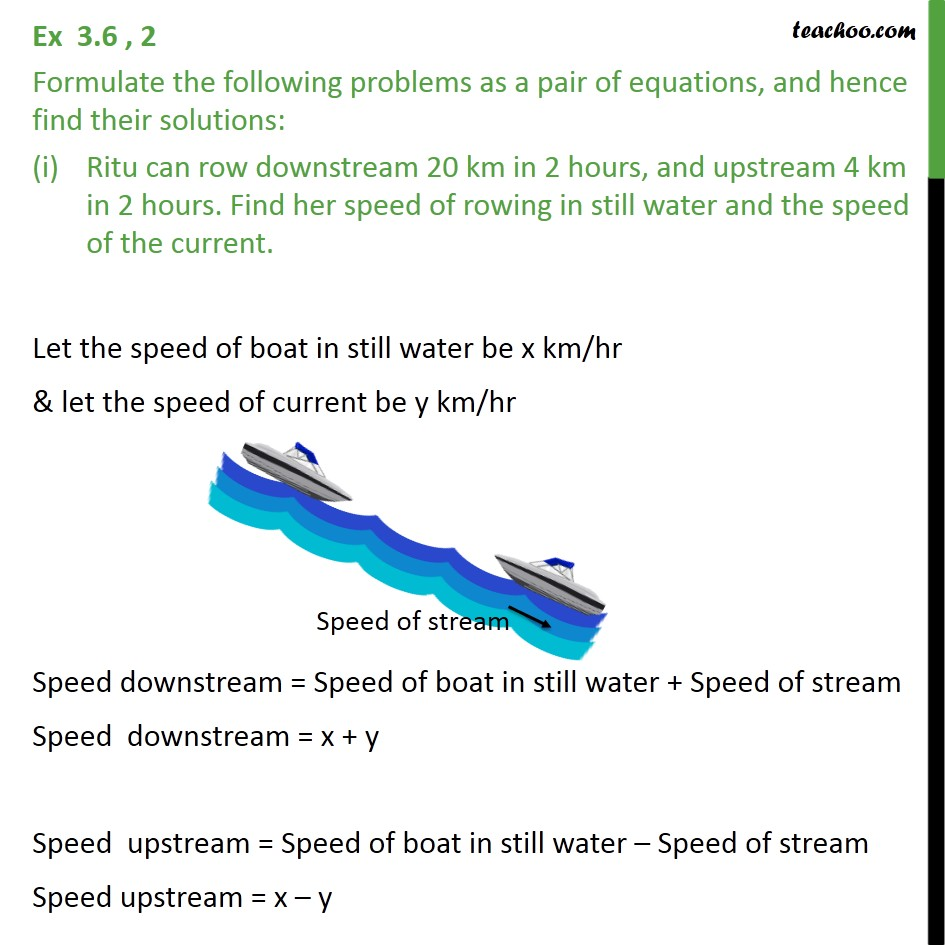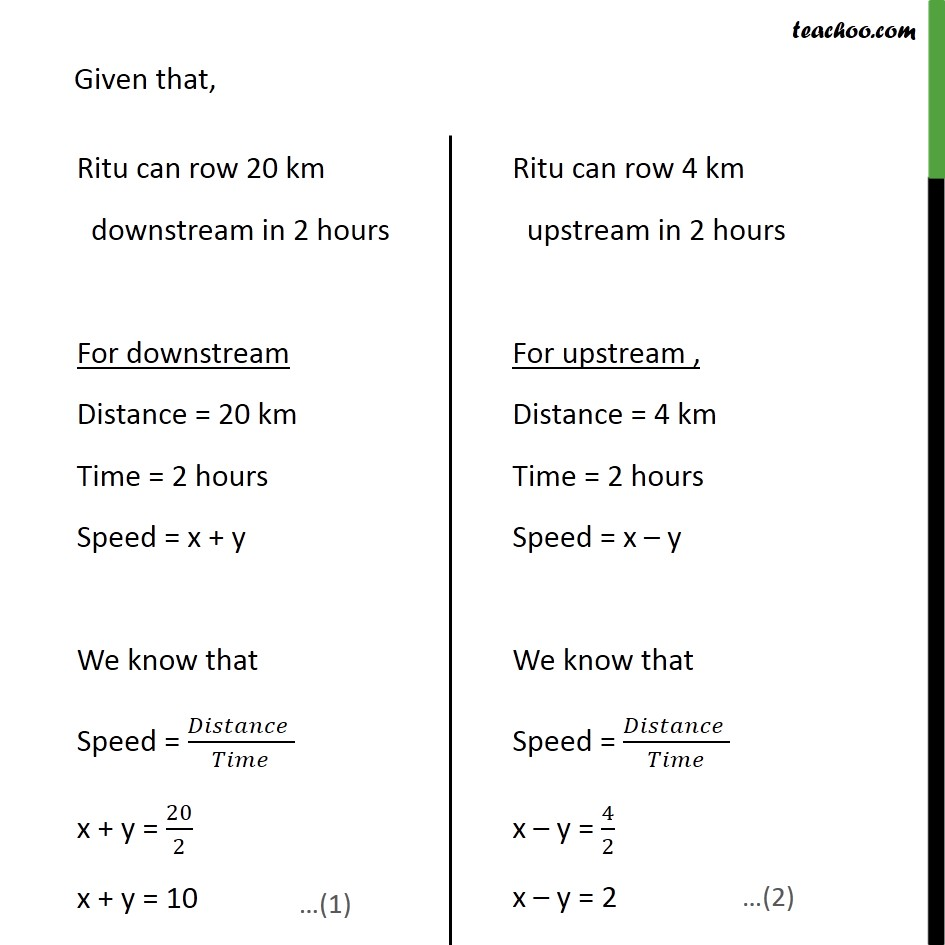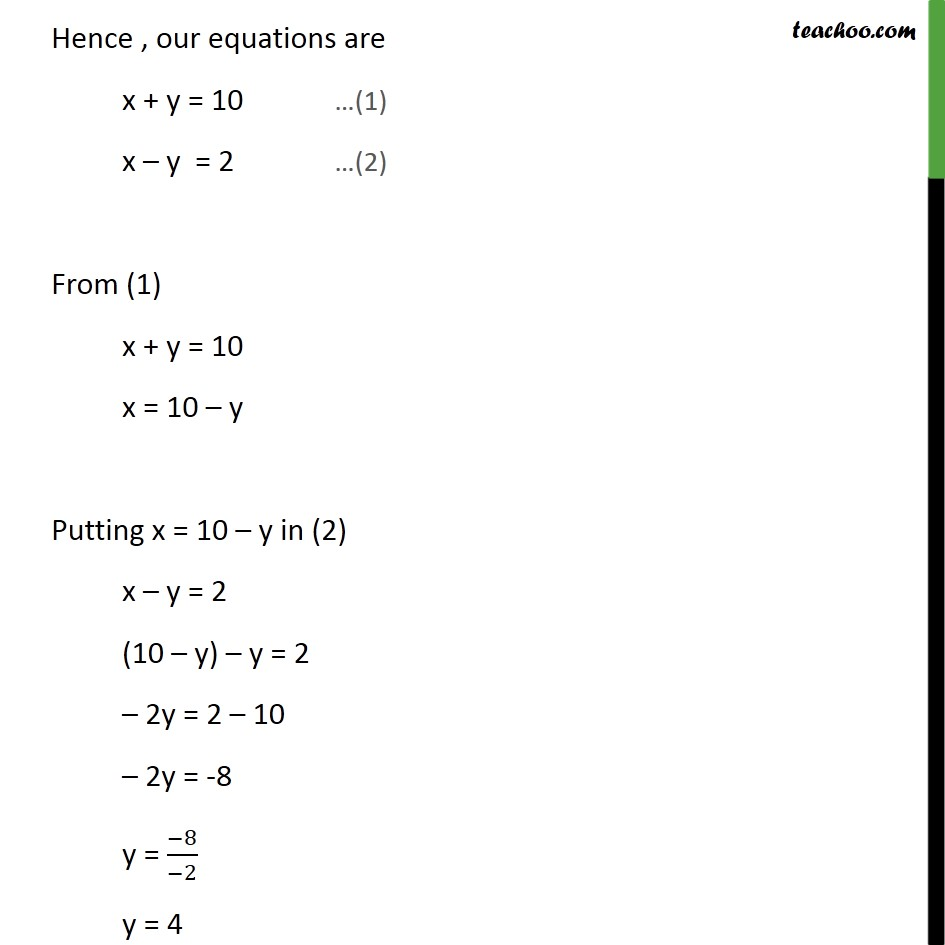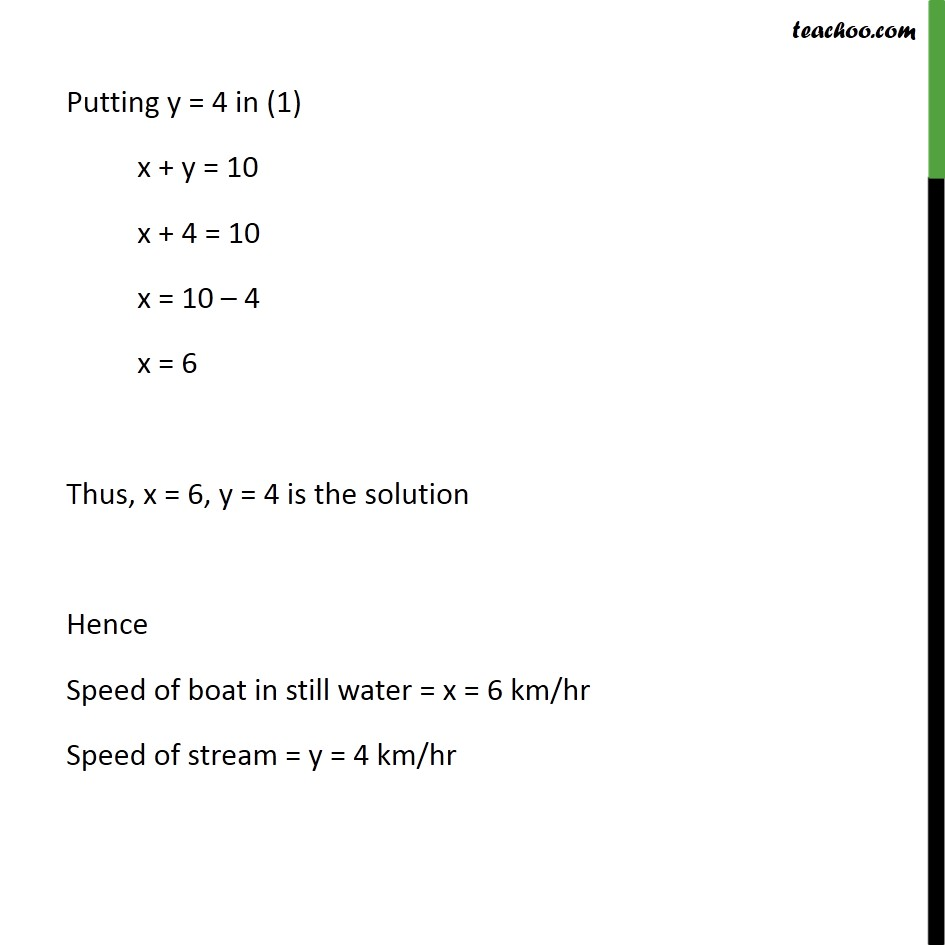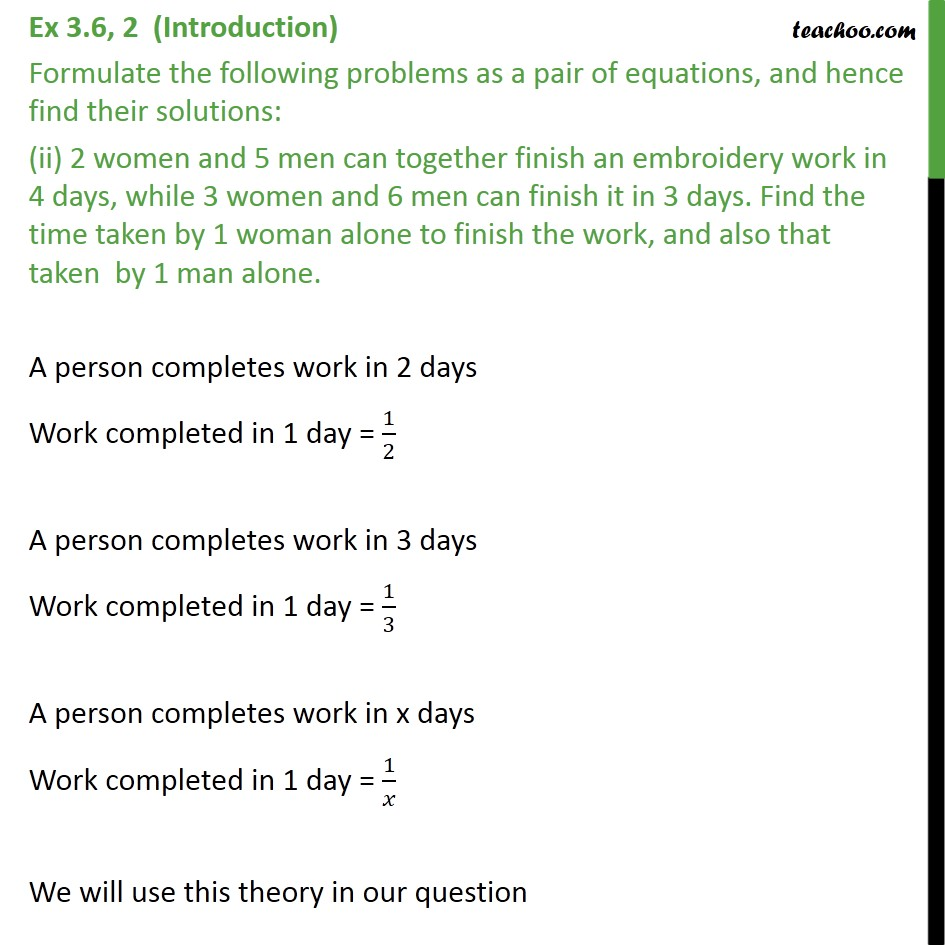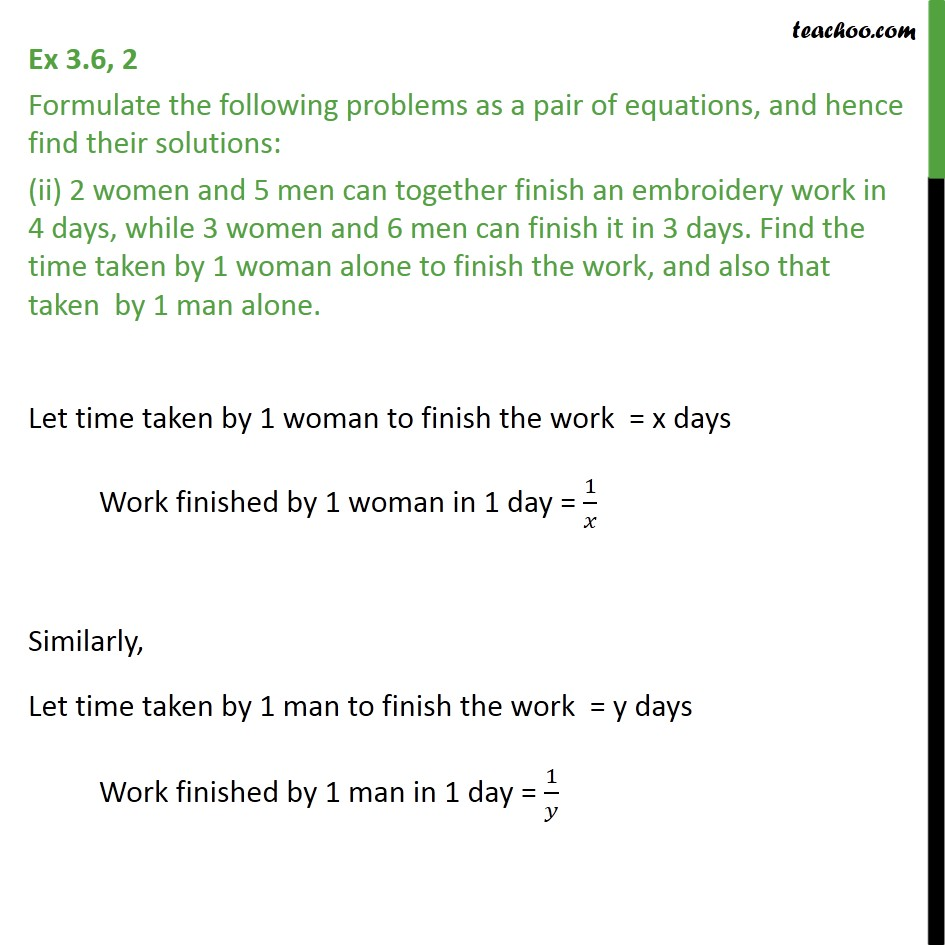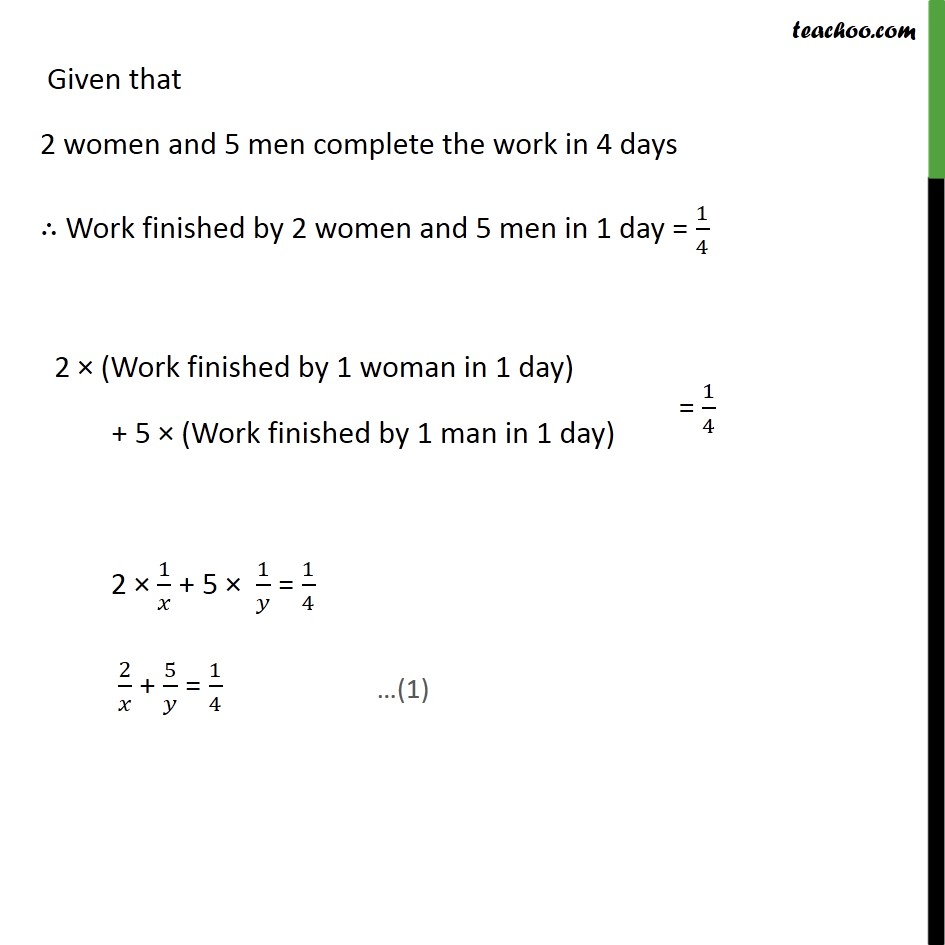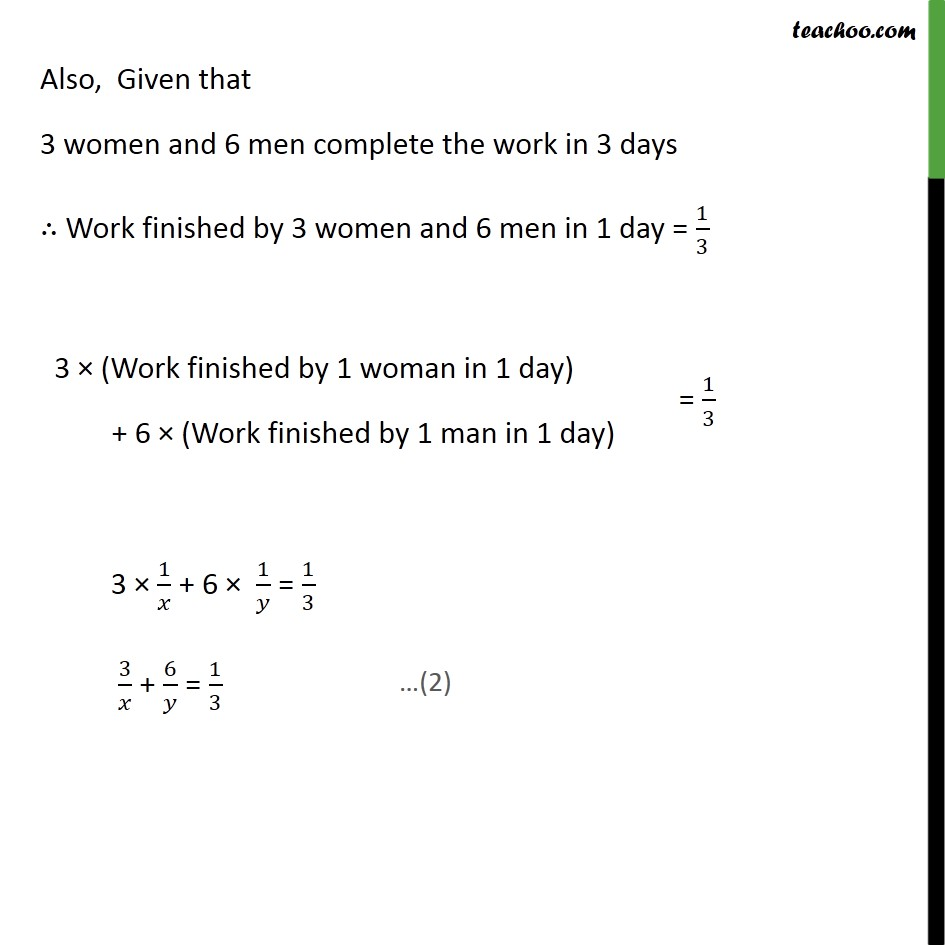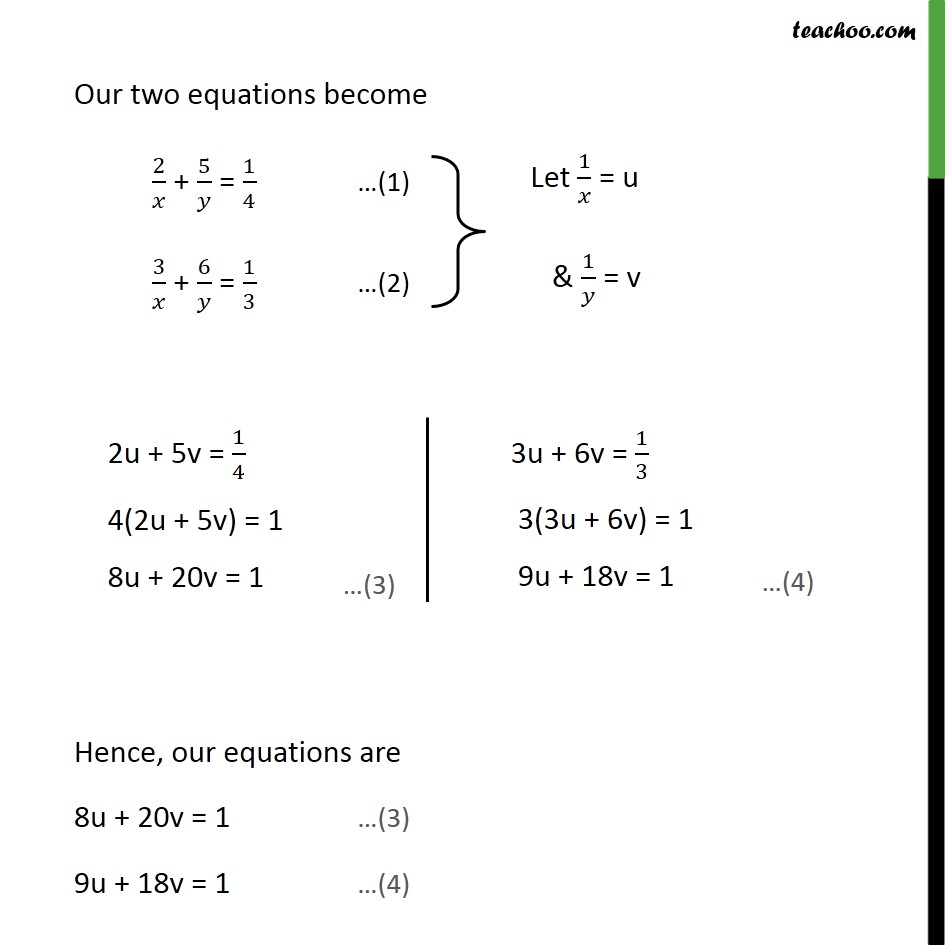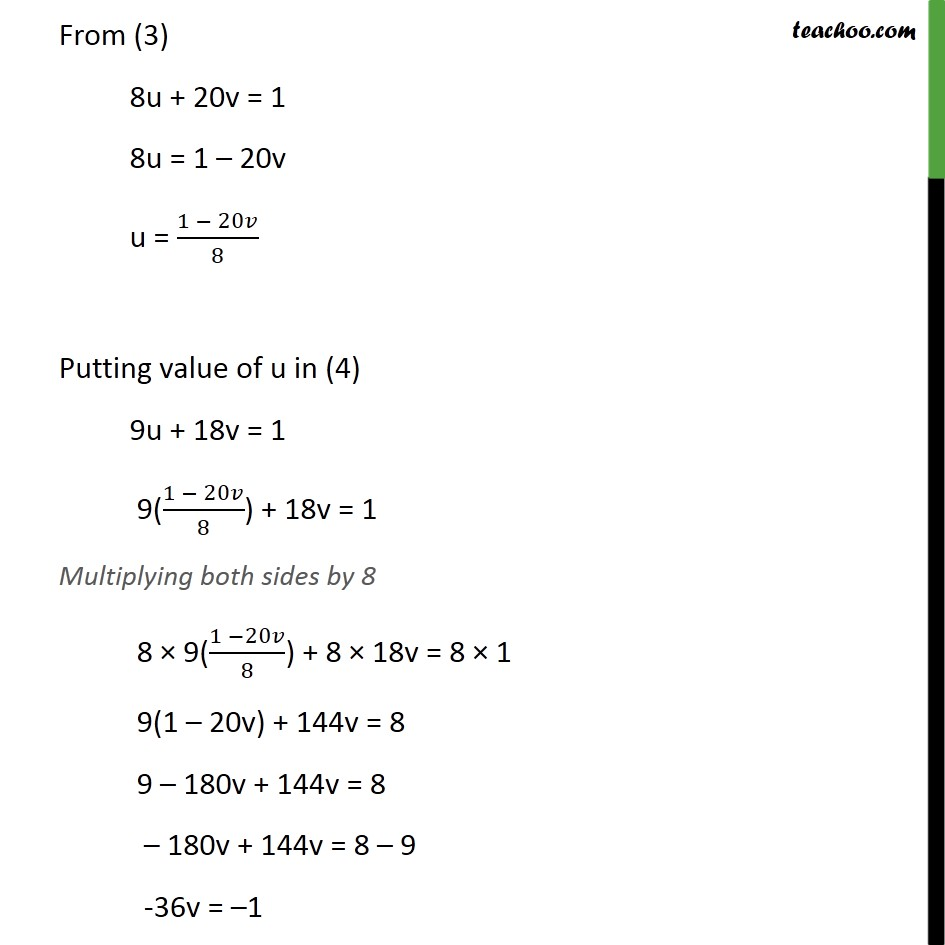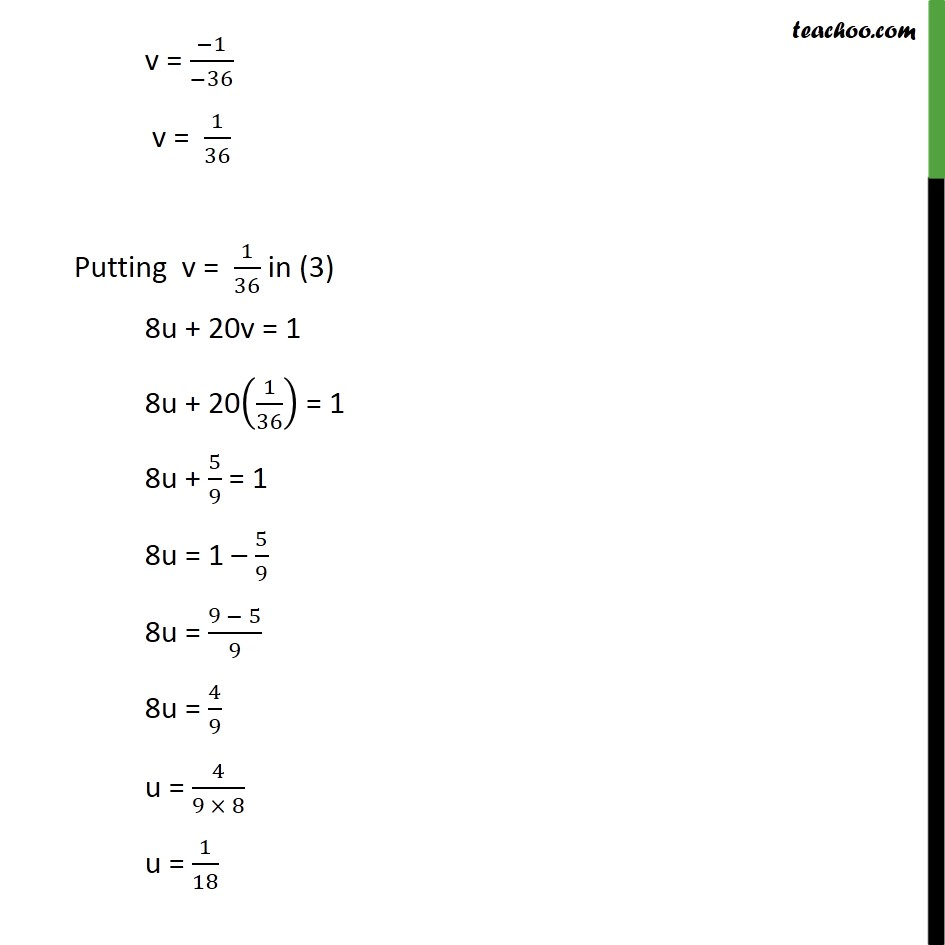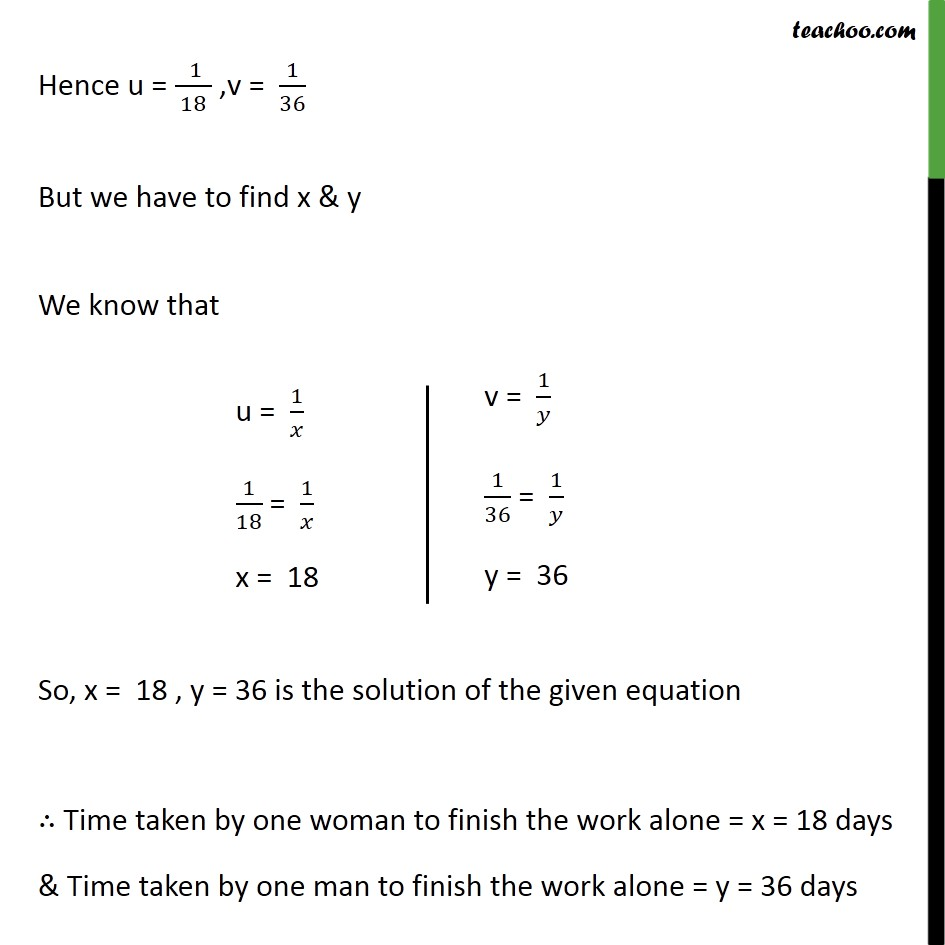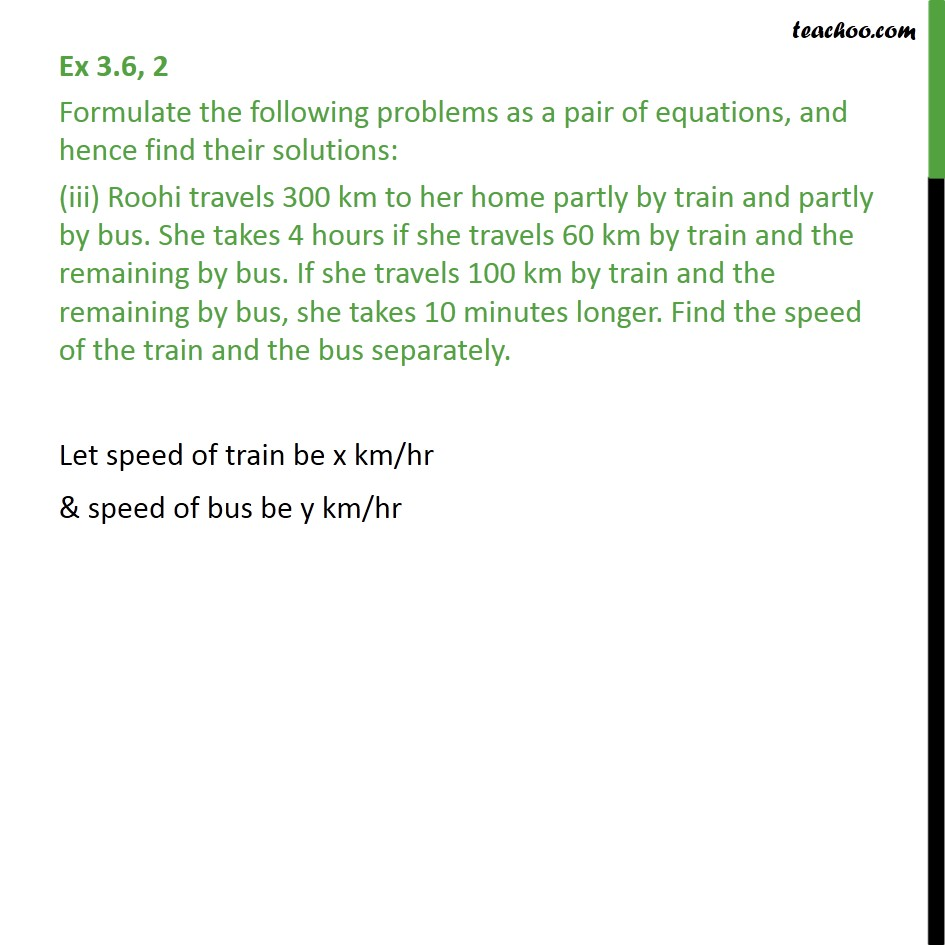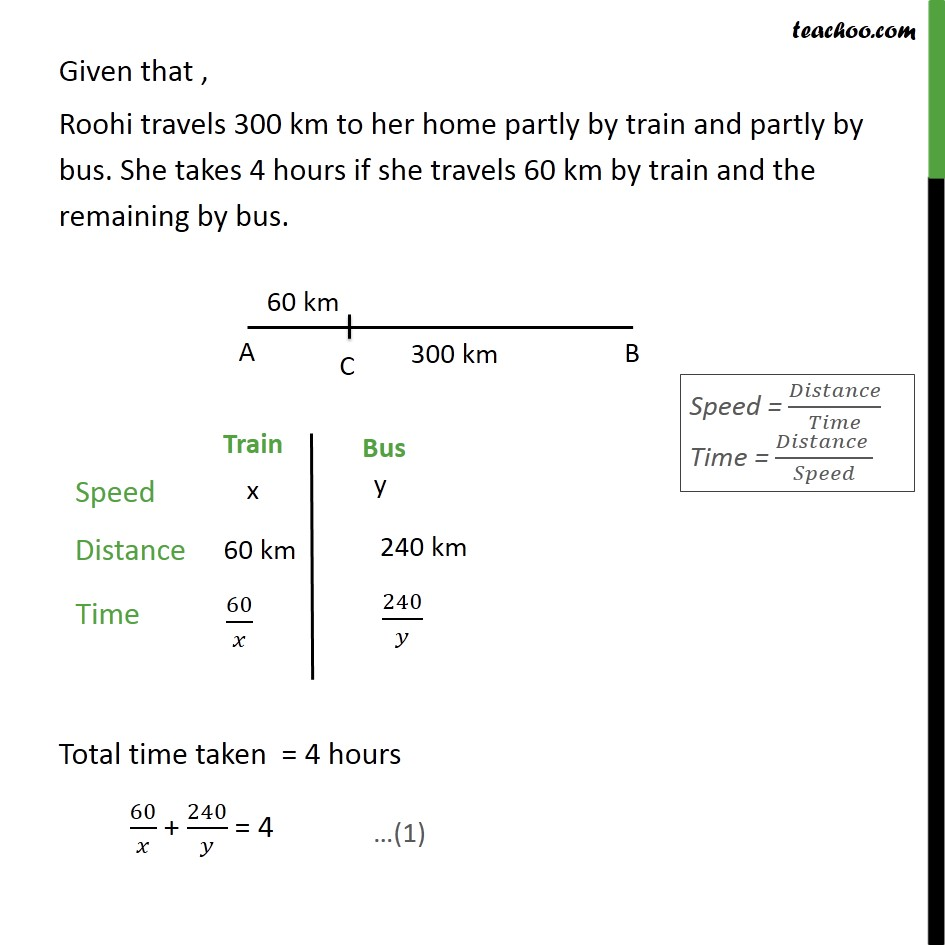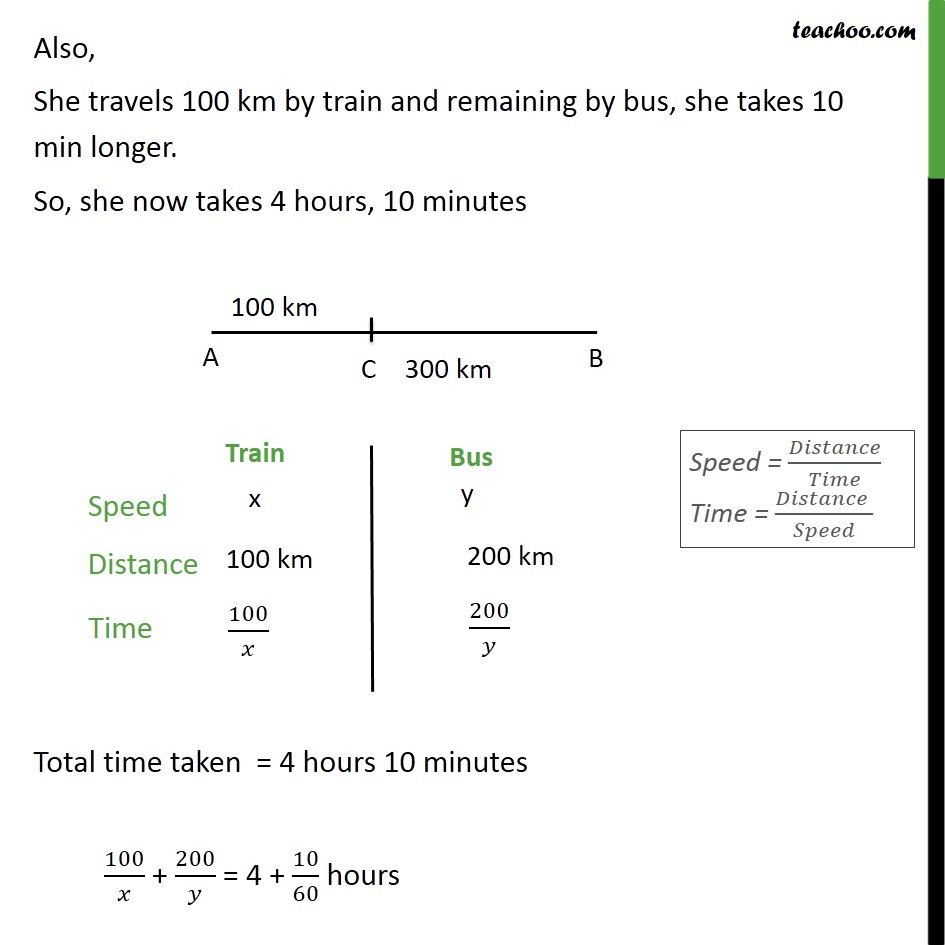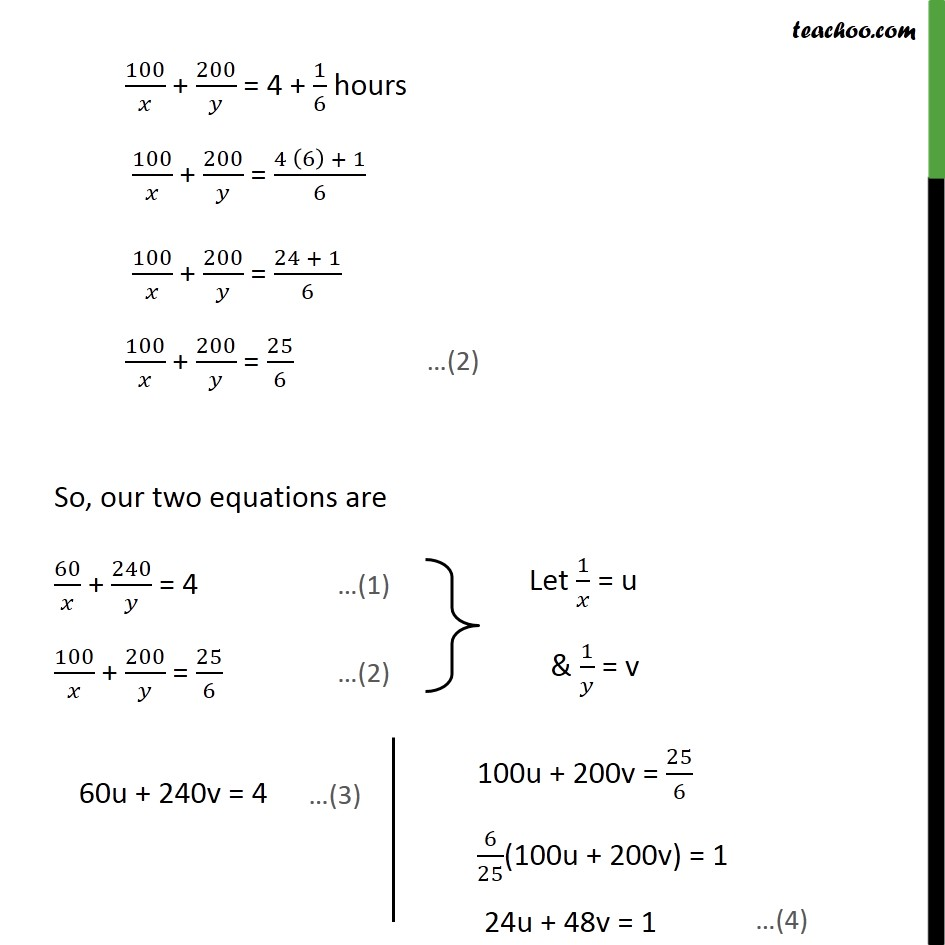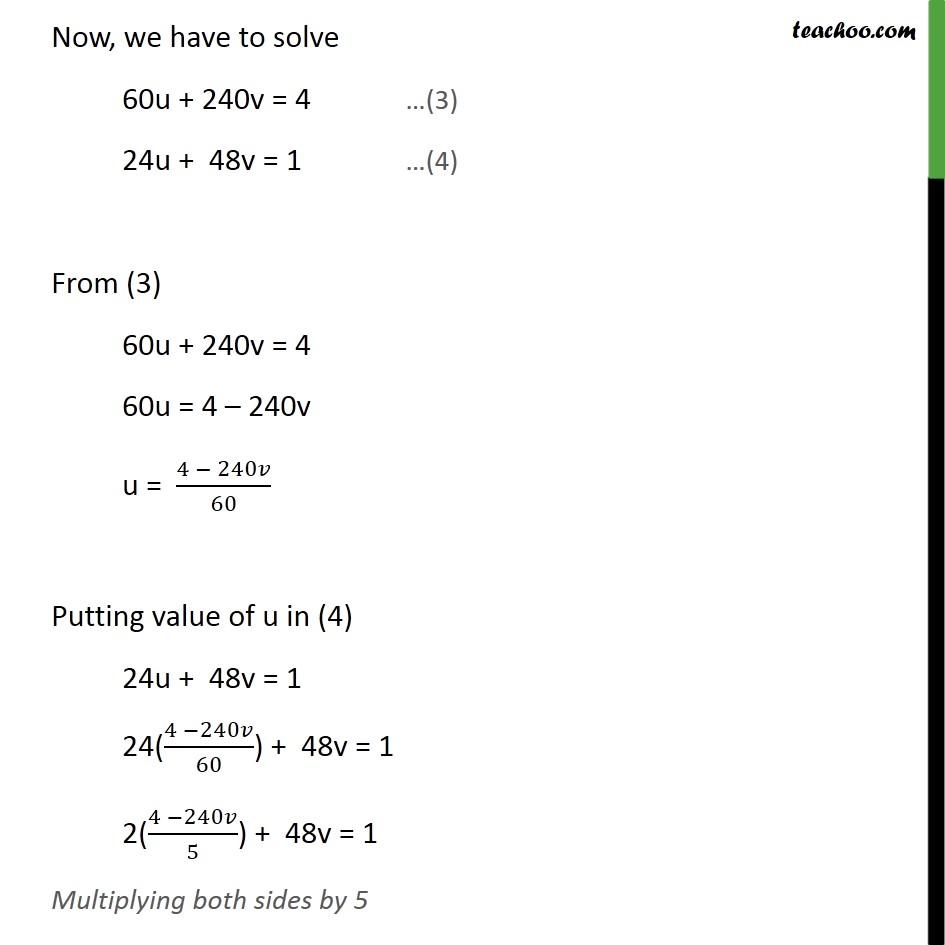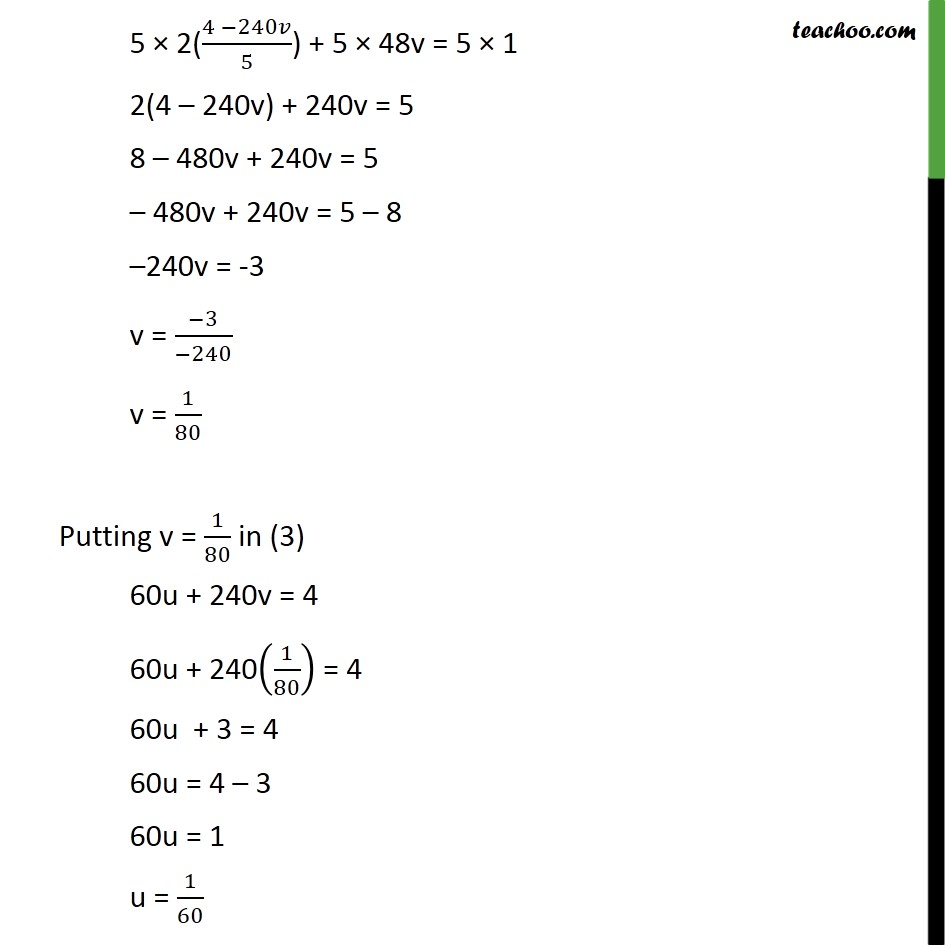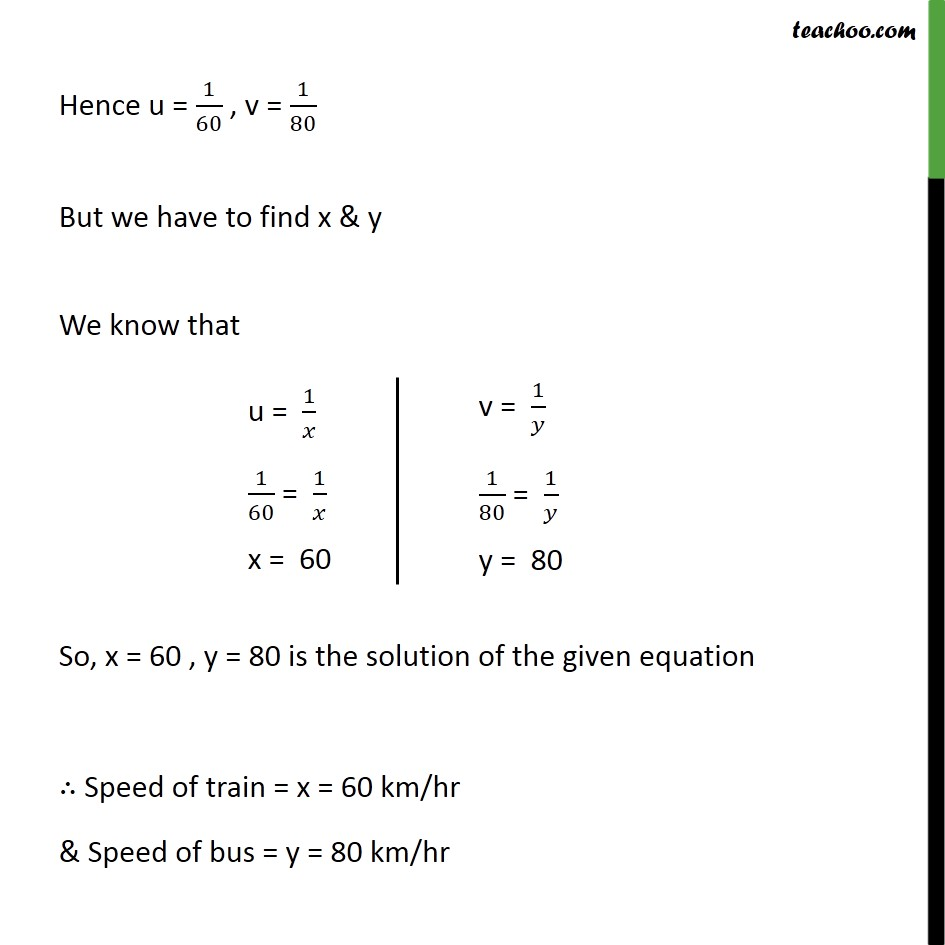1. Chapter 3 Class 10 Pair of Linear Equations in Two Variables
2. Concept wise
3. Mix questions - Equation to be formed

Transcript

Ex 3.6 , 2 Formulate the following problems as a pair of equations, and hence find their solutions: Ritu can row downstream 20 km in 2 hours, and upstream 4 km in 2 hours. Find her speed of rowing in still water and the speed of the current. Let the speed of boat in still water be x km/hr & let the speed of current be y km/hr Speed downstream = Speed of boat in still water + Speed of stream Speed downstream = x + y Speed upstream = Speed of boat in still water Speed of stream Speed upstream = x y Given that, Hence , our equations are x + y = 10 (1) x y = 2 (2) From (1) x + y = 10 x = 10 y Putting x = 10 y in (2) x y = 2 (10 y) y = 2 2y = 2 10 2y = -8 y = ( 8)/( 2) y = 4 Putting y = 4 in (1) x + y = 10 x + 4 = 10 x = 10 4 x = 6 Thus, x = 6, y = 4 is the solution Hence Speed of boat in still water = x = 6 km/hr Speed of stream = y = 4 km/hr Ex 3.6, 2 (Introduction) Formulate the following problems as a pair of equations, and hence find their solutions: (ii) 2 women and 5 men can together finish an embroidery work in 4 days, while 3 women and 6 men can finish it in 3 days. Find the time taken by 1 woman alone to finish the work, and also that taken by 1 man alone. A person completes work in 2 days Work completed in 1 day = 1/2 A person completes work in 3 days Work completed in 1 day = 1/3 A person completes work in x days Work completed in 1 day = 1/ We will use this theory in our question Ex 3.6, 2 Formulate the following problems as a pair of equations, and hence find their solutions: (ii) 2 women and 5 men can together finish an embroidery work in 4 days, while 3 women and 6 men can finish it in 3 days. Find the time taken by 1 woman alone to finish the work, and also that taken by 1 man alone. Let time taken by 1 woman to finish the work = x days Work finished by 1 woman in 1 day = 1/ Similarly, Let time taken by 1 man to finish the work = y days Work finished by 1 man in 1 day = 1/ Given that 2 women and 5 men complete the work in 4 days Work finished by 2 women and 5 men in 1 day = 1/4 2 (Work finished by 1 woman in 1 day) + 5 (Work finished by 1 man in 1 day) 2 1/ + 5 1/ = 1/4 2/ + 5/ = 1/4 Also, Given that 3 women and 6 men complete the work in 3 days Work finished by 3 women and 6 men in 1 day = 1/3 3 (Work finished by 1 woman in 1 day) + 6 (Work finished by 1 man in 1 day) 3 1/ + 6 1/ = 1/3 3/ + 6/ = 1/3 Our two equations become 2/ + 5/ = 1/4 (1) 3/ + 6/ = 1/3 (2) Hence, our equations are 8u + 20v = 1 (3) 9u + 18v = 1 (4) From (3) 8u + 20v = 1 8u = 1 20v u = (1 20 )/8 Putting value of u in (4) 9u + 18v = 1 9((1 20 )/8) + 18v = 1 Multiplying both sides by 8 8 9((1 20 )/8) + 8 18v = 8 1 9(1 20v) + 144v = 8 9 180v + 144v = 8 180v + 144v = 8 9 -36v = 1 v = ( 1)/( 36) v = 1/36 Putting v = 1/36 in (3) 8u + 20v = 1 8u + 20(1/36) = 1 8u + 5/9 = 1 8u = 1 5/9 8u = (9 5)/9 8u = 4/9 u = 4/(9 8) u = 1/18 Hence u = ( 1)/( 18 ) ,v = 1/36 But we have to find x & y We know that So, x = 18 , y = 36 is the solution of the given equation Time taken by one woman to finish the work alone = x = 18 days & Time taken by one man to finish the work alone = y = 36 days Ex 3.6, 2 Formulate the following problems as a pair of equations, and hence find their solutions: (iii) Roohi travels 300 km to her home partly by train and partly by bus. She takes 4 hours if she travels 60 km by train and the remaining by bus. If she travels 100 km by train and the remaining by bus, she takes 10 minutes longer. Find the speed of the train and the bus separately. Let speed of train be x km/hr & speed of bus be y km/hr Given that , Roohi travels 300 km to her home partly by train and partly by bus. She takes 4 hours if she travels 60 km by train and the remaining by bus. Total time taken = 4 hours 60/ + 240/ = 4 Also, She travels 100 km by train and remaining by bus, she takes 10 min longer. So, she now takes 4 hours, 10 minutes Total time taken = 4 hours 10 minutes 100/ + 200/ = 4 + 10/60 hours 100/ + 200/ = 4 + 1/6 hours 100/ + 200/ = (4 (6) + 1)/6 100/ + 200/ = (24 + 1)/6 100/ + 200/ = 25/6 So, our two equations are 60/ + 240/ = 4 (1) 100/ + 200/ = 25/6 (2) Now, we have to solve 60u + 240v = 4 (3) 24u + 48v = 1 (4) From (3) 60u + 240v = 4 60u = 4 240v u = (4 240 )/60 Putting value of u in (4) 24u + 48v = 1 24((4 240 )/60) + 48v = 1 2((4 240 )/5) + 48v = 1 Multiplying both sides by 5 5 2((4 240 )/5) + 5 48v = 5 1 2(4 240v) + 240v = 5 8 480v + 240v = 5 480v + 240v = 5 8 240v = -3 v = ( 3)/( 240) v = 1/80 Putting v = 1/80 in (3) 60u + 240v = 4 60u + 240(1/80) = 4 60u + 3 = 4 60u = 4 3 60u = 1 u = 1/60 Hence u = 1/60 , v = 1/80 But we have to find x & y We know that So, x = 60 , y = 80 is the solution of the given equation Speed of train = x = 60 km/hr & Speed of bus = y = 80 km/hr

Mix questions - Equation to be formed